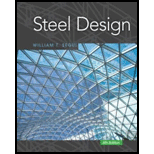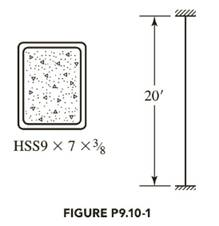# An H S S 9 × 7 × 3 8 filled with concrete is used as a composite column, as shown in Figure P9.10-1. The steel has a yield stress of F y = 46 ksi, and the concrete has a compressive strength of f c ' = 4 ksi. Compute the nominal strength of the column.### Steel Design (Activate Learning wi...

6th Edition
Segui + 1 other
Publisher: Cengage Learning
ISBN: 9781337094740

#### Solutions

Chapter
Section### Steel Design (Activate Learning wi...

6th Edition
Segui + 1 other
Publisher: Cengage Learning
ISBN: 9781337094740
Chapter 9, Problem 9.10.1P
Textbook Problem
1 views

## An H S S 9 × 7 × 3 8 filled with concrete is used as a composite column, as shown in Figure P9.10-1. The steel has a yield stress of F y = 46 ksi, and the concrete has a compressive strength of f c ' = 4 ksi. Compute the nominal strength of the column.To determine

The nominal strength of the column.

### Explanation of Solution

Given:

HSS9×7×38Fy=46ksifc'=4ksi

Formula used:

Pno=FyAs+FysrAsr+f'cAc

Where, Fy is yield stress of the steel shape.

As is cross-sectional area of the steel shape.

Fysr is yield stress of the longitudinal reinforcing steel bars.

Asr is cross-sectional area of the reinforcing steel.

Pe=π2(EI)eff(KL)2

Where, Pe is the Euler buckling load.

(EI)eff is the effective flexural rigidity of the composite section.

(EI)eff=EsIs+0.5EsIsr+C1EcIc

Is is moment of inertia of the steel shape about the axis of buckling.

Isr is moment of inertia of the longitudinal reinforcing bars about the axis ofbuckling.

Ic is moment of inertia of the concrete section about the elastic neutral axis of the compositesection.

Calculation:

The following dimensions and properties from Part 1 of the Manual will be needed: For an HSS9×7×38,

As=10.4in.2t(des)=0.349in.Iy=80.4in.4

As is cross-sectional area of member.

t(des) is design wall thickness.

Iy is moment of inertia of member taken about Y-axis.

Ec=w1.5fc'=(145)1.54=3492ksi

The area of concrete Ac can be estimated as

bdAs

b=7in.d=9in.

b is width of member

d is depth of member parallel to Y-axis

bdAs=7(9)10.4=52.6in.2

or more accurately as follows:

The corner radius is given in Part 1 of the Manual as 2 t(des). The area of a quarter-circle spandrel with a radius r is

r2πr24=(2t)2π(2t)24=t2(4π)=0.3492(4π)=0.105in.2

And since there are four of these segments to be deducted from the area bdAs,

Ac=bdAs4(0.105)=7(9)10.44(0.105)=52.18in.4

For computing the moment of inertia of the concrete, the moment of inertia of the spandrel about an axis parallel to the 9-inch side through the point of tangency is

I=(13π16)r4=(13π16)(2t)4=(163π)t4=(163π)(0

### Still sussing out bartleby?

Check out a sample textbook solution.

See a sample solution

#### The Solution to Your Study Problems

Bartleby provides explanations to thousands of textbook problems written by our experts, many with advanced degrees!

Get Started

Find more solutions based on key concepts
A machinist in an engineering machine shop has ordered a sheet of plastic with dimensions of 10 ft 12 ft 1 in...

Engineering Fundamentals: An Introduction to Engineering (MindTap Course List)

What is a correlated subquery? Give an example.

Database Systems: Design, Implementation, & Management

Name the two methods of attaching brake lining materials to the brake shoes.

Automotive Technology: A Systems Approach (MindTap Course List)

Describe the purpose of a CAPTCHA.

Enhanced Discovering Computers 2017 (Shelly Cashman Series) (MindTap Course List)

If your motherboard supports ECC DDR3 memory, can you substitute non-ECC DDR3 memory?

A+ Guide to Hardware (Standalone Book) (MindTap Course List)

List some applications that use high-pressure piping.

Welding: Principles and Applications (MindTap Course List)